# Jähne test pattern – take 3

Earlier today I told you that I was feeling a little dense because I couldn't figure out the right parameters to use in the tanh term of this test pattern: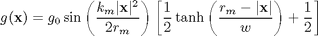(This is equation 10.63 in Practical Handbook on Image Processing for Scientific Applications by Bernd Jahne.)

I'm grateful to reader Alex H for quickly enlightening me. He described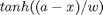as an approximation to a step function, where a is the location of the step and w is the width of the transition. That was very helpful.

I've also realized that I misinterpreted the meaning of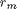. In the book this is described as the "maximum radius of the pattern," and I assumed this would be fixed as the distance from the center of the square image to one of its corners. But now I realize that the author intended for this to be an adjustable parameter. That is, one can setso that the maximum instantaneous frequency is reached closer to the center than at the image corners.

For example, I can set the parameters so that the maximum instantaneous frequency ofis reached in the center of the image edges, and then the tapering function prevents aliasing artifacts from appearing as you move out to the corners. The book's figure 10.23 is based on maximum instantaneous frequency of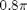(a period of 2.5 samples) reached at the edges, so I'll use that.

[x,y] = meshgrid(-200:200);
km = 0.8*pi;
rm = 200;
w = rm/10;
term1 = sin( (km * r.^2) / (2 * rm) );
term2 = 0.5*tanh((rm - r)/w) + 0.5;
g = term1 .* term2;
imshow(g,[])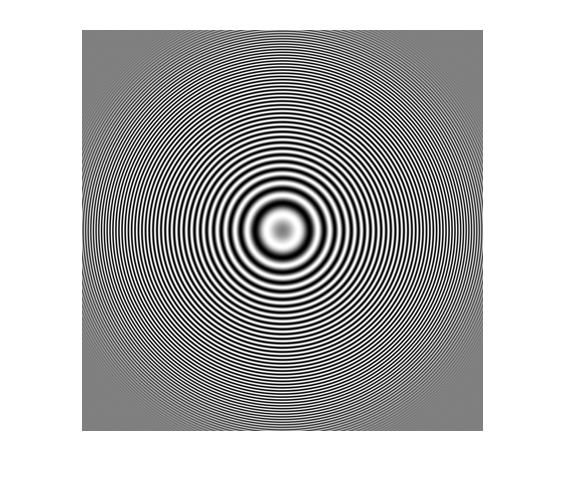Finally, a result that looks like the figure in the book!

Thanks again, Alex.

Published with MATLAB® 7.12

|